# Fundamental frequencies of square wave and sine wave

galaxy_twirl

## Homework Statement

What are the fundamental frequencies for a 50 kHz square waveform of 50% duty cycle and a 25 kHz sinusoidal waveform, respectively? (The duty cycle of a square waveform is the ratio between the pulse duration and the pulse period.)

## Homework Equations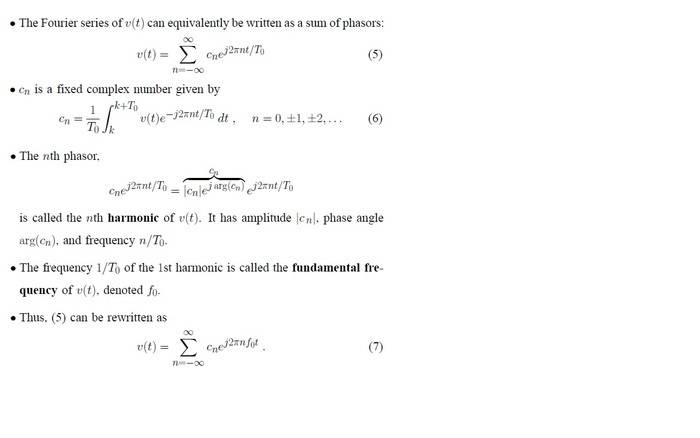My teacher then gave an example to illustrate v(t) and cn. Hence, I have a feeling that I should use these formula to find the answer.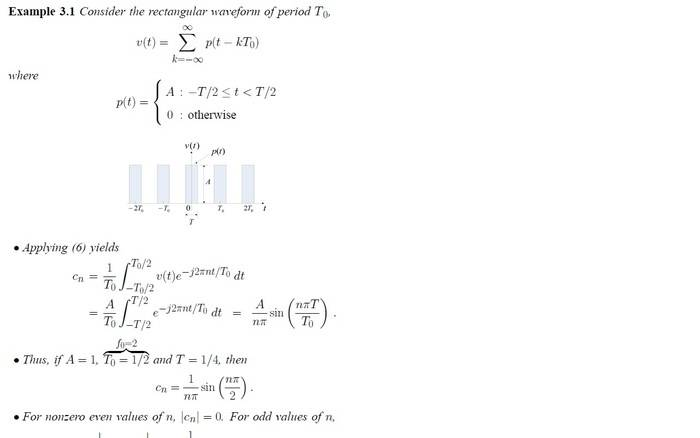## The Attempt at a Solution

I am at a loss of which equation to use because there are too many representations of v(t) and cn in my lecture notes. Nevertheless, I shall attempt o solve the above question.

For the square wave, with its frequency at 50kHz, which is f0, its period, 1/f0 is 2X10-5 seconds. I don't understand what does T stand for in the equation, but I assume it refres to the amount of time the signal was turned on. Since the signal is ON for half the time, T will be 1X10-5.

Hence, I will have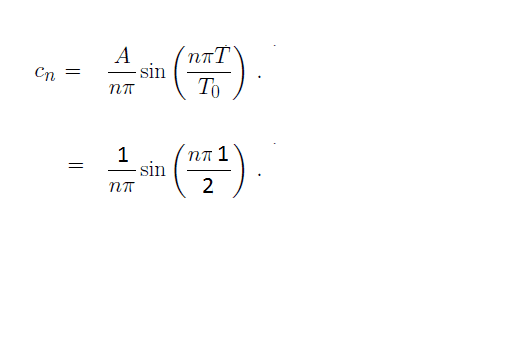Am I correct?

May I have some hints as to how to start the question for the part on sine wave?

Thank you everyone. :)

## Answers and Replies

Mentor
The question asks for the fundamental frequencies of the given waveforms, one a square wave and the other a sine wave. You should be able to get to the answers without any math at all :)

The Fourier series represents a sum of frequency components, all of which are sinusoidal. In effect it breaks down a given periodic waveform into a series of sinusoidal frequency components.

A pure sine wave has only one component frequency, namely that of the sine wave itself. So you should be able to dispose of the 25 KHz sinewave easily enough.

The square wave can also be dealt with by inspection if you picture the largest sinewave that will fit its outline.

galaxy_twirl
Hi gneill. Thank you for your help! :)

Ah I see. I determined the f0 in the lab by feeding in sinusoidal signals, square pulses and triangular waves into the FFT (Fast Fourier Transform) Oscilloscope and they all turned out to match the frequencies supplied by the signal generator regardless of the duty cycle. :)

The square wave can also be dealt with by inspection if you picture the largest sinewave that will fit its outline.

I know from my lecture notes that a sine wave can look like a square wave with squiggles when n becomes a larger number in sin(nx), as seen below: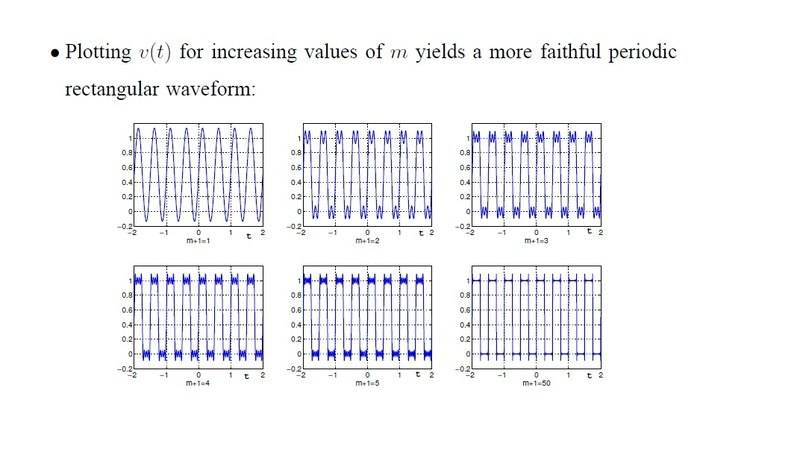Do you mean the above in your reply to my question on square wave? :) Just wondering, how do you know how large should the sine wave get into order to achieve a square waveform?

Thanks again! :D

Mentor
Do you mean the above in your reply to my question on square wave? :) Just wondering, how do you know how large should the sine wave get into order to achieve a square waveform?

I meant that by looking at a given square wave you should be able to see the period (or frequency) of the fundamental sine wave that fits its shape: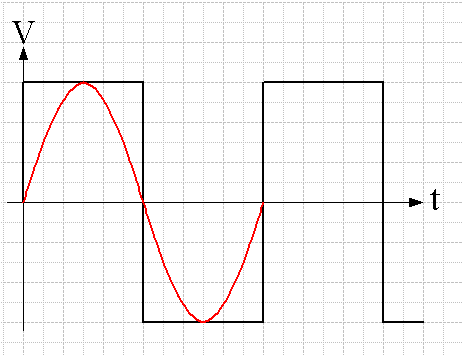Determining the magnitude of that fundamental frequency for the Fourier series of the square wave is another matter :)

•galaxy_twirl
galaxy_twirl
Ah I see.

Thank you! :D